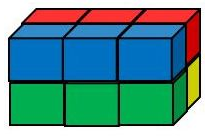10.8 Exploring Surface Area and Volume Activity

# Materials

• 12 small cubes (1 cm3 suggested) per group of students (12 cubes per student if possible)
• Paper
• Pencil

# Directions

Students will explore how rearranging cubes to construct a shape will affect the surface area and volume of the shape.

• Students will work in small groups or partners.
• Each group should get at least one set of 12 small cubes.
• Ask students to stack cubes to create a rectangular prism using all 12 cubes.
• Have students record the pattern you arranged.
• Students should count the surface area of the prism you created and record it.
• Discuss surface area with class, defining it and showing the example they counted.
• Students should count the volume of the prism you created and record it.
• Discuss volume with the class, defining it and showing the example they counted.
• Have students re-arrange the cubes to construct a different rectangular prism using all 12 cubes. If the group has access to more than one set of cubes, leave the original prism visible.
• Students should count the surface area of the new prism and record it.
• Students should count the volume of the new prism and record it.
• Groups should compare the surface area of the two designs.
• Groups should compare the volume of the two designs.
• Be sure to note that the surface area likely changed with the new design, but the amount of space (volume) of their prism will remain the same since it is constructed with the same number of cubes.
• Challenge - Create a prism with the smallest surface area.
• Challenge - Create a prism with the largest surface area.

This activity can be expanded with 24 cubes available to each group.